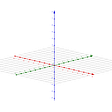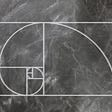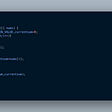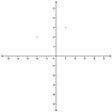# Leetcode 354: Russian Doll Envelopes

`Input: [[5,4],[6,4],[6,7],[2,3]]Output: 3 Explanation: The maximum number of envelopes you can Russian doll is 3 ([2,3] => [5,4] => [6,7]).`
`class Solution {public:    int maxEnvelopes(vector<vector<int>>& envelopes) {        if (envelopes.size() == 0) return 0;                sort(envelopes.begin(), envelopes.end(), [](const vector<int>& lhs, const vector<int>& rhs) {            return lhs.front() < rhs.front() || (lhs.front() == rhs.front() && lhs.back() < rhs.back());        });        vector<int> dp(envelopes.size(), 1);        int res = 1;        for (int i = 1; i < dp.size(); ++i) {            for (int j = 0; j < i; ++j) {                if (envelopes[j] < envelopes[i] && envelopes[j] < envelopes[i]) {                    dp[i] = max(dp[i], dp[j] + 1);                    res = max(dp[i], res);                }            }        }                return res;    }};`
`class Solution {public:  int maxEnvelopes(vector<pair<int, int>>& envelopes) { int len = envelopes.size(); if (len == 0) return 0;sort(envelopes.begin(), envelopes.end(),    [](pair<int, int>& a, pair<int, int>& b) {     return a.first < b.first || (a.first == b.first && a.second > b.second);   });vector<int> dp; for (auto &item: envelopes) {   auto search = lower_bound(dp.begin(), dp.end(), item.second);   if (search == dp.end()) dp.push_back(item.second);   else if (*search > item.second) *search = item.second; }return dp.size();  }};`

--

--

--

## More from dume0011

Love podcasts or audiobooks? Learn on the go with our new app.

## Multi-Dimensional Arrays## Collection Views with Compositional Layout## Have you lost your AppEngine default service account and the world went dark?## A Short Introduction into Julia## Depth / Breath First Search Matrix Traversal in Python with Interactive Code [ Back to Basics ]## X-Launcher and Synaps KYC## High Level Synthesis using Random Forest Algorithm## Largest Sum Contiguous Sub-Array## [Leetcode] K Closest Points to Origin## Binary Tree Threading & Morris In-order Traversal